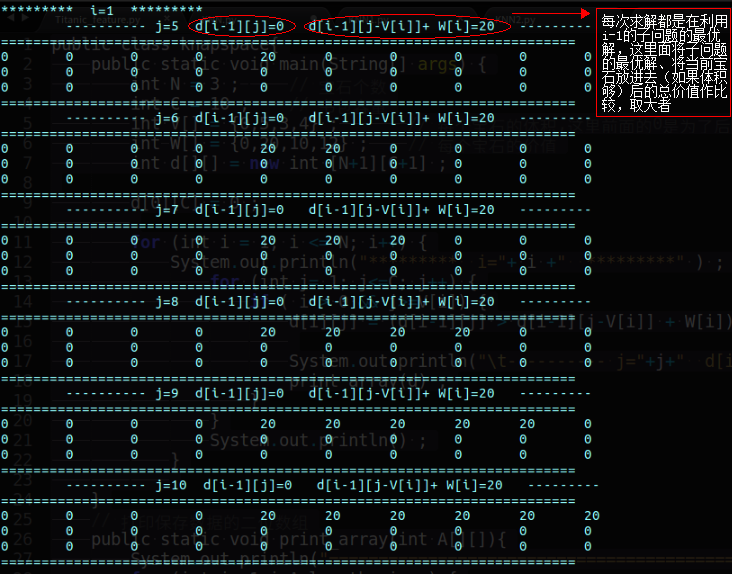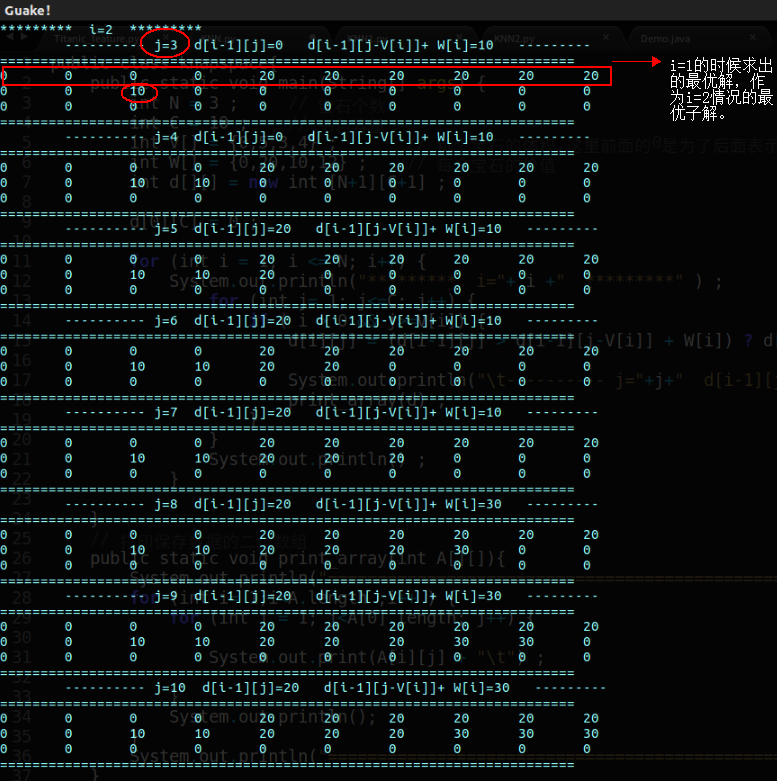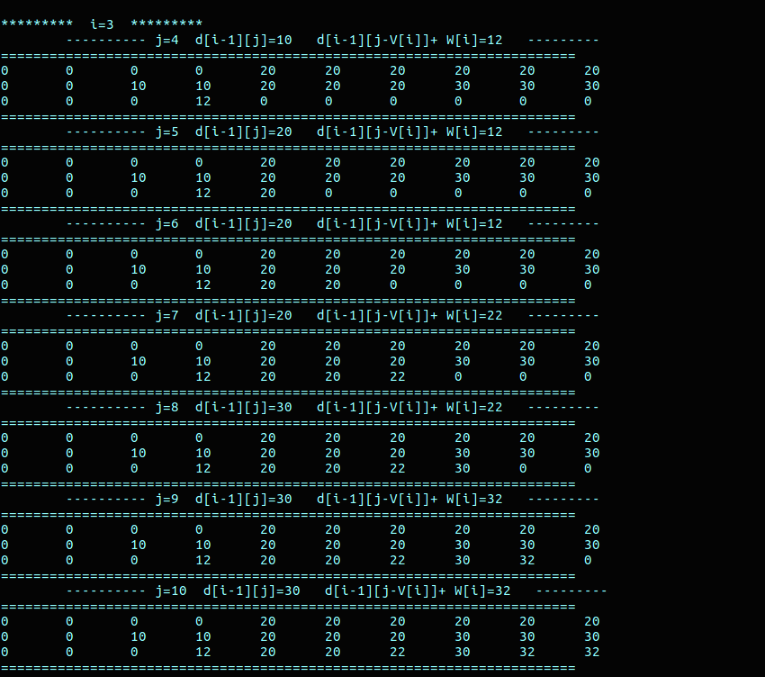# 一步一步看清动态规划----背包问题（java解）

———————————更新——————————–

———————————原文——————————–

# 题目背景

OK，如果是你，你会怎么做？你斩钉截铁的说：动态规划啊！恭喜你，答对了。 那么让我们来看看，动态规划中最最最重要的两个概念： 状态和状态转移方程在这个问题中分别是什么。

# 程序及详细讲解


public class knapspace{
public static void main(String[] args) {
int N = 3 ;     // 宝石个数
int C = 10 ;    // 书包容量
int V[] = {0,5,3,4} ;       // 每个宝石的体积，这里前面的0是为了后面表示方便，即V表示为第一个宝石的体积，下同
int W[] = {0,20,10,12} ;    // 每个宝石的价值
int d[][] = new int [N+1][C+1] ;

d[C] = 0 ;

for (int i = 1; i <= N; i++) {
for (int j= 1; j<=C; j++) {
if ( i > 0 && j>=V[i]) {
// 状态转移方程
d[i][j] = (d[i-1][j] > d[i-1][j-V[i]] + W[i]) ? d[i-1][j] : d[i-1][j-V[i]] + W[i] ;
}
}
}
System.out.println(d[N][C]) ;
}
}

public class knapspace{
public static void main(String[] args) {
int N = 3 ;     // 宝石个数
int C = 10 ;    // 书包容量
int V[] = {0,5,3,4} ;       // 每个宝石的体积,这里前面的0是为了后面表示方便，即V表示为第一个宝石的体积，下同
int W[] = {0,20,10,12} ;    // 每个宝石的价值
int d[][] = new int [N+1][C+1] ;    // 状态数组

d[C] = 0 ;

for (int i = 1; i <= N; i++) {
System.out.println("*********  i="+ i +"  *********" ) ;
for (int j= 1; j<=C; j++) {
if ( i > 0 && j>=V[i]) {
// 状态转移方程
d[i][j] = (d[i-1][j] > d[i-1][j-V[i]] + W[i]) ? d[i-1][j] : d[i-1][j-V[i]] + W[i] ;

System.out.println("\t---------- j="+j+"  d[i-1][j]="+d[i-1][j]+"   d[i-1][j-V[i]]+ W[i]="+(d[i-1][j-V[i]]+ W[i])+"   ---------") ;
// 打印每次保存状态的数组
print_array(d) ;
}
}
System.out.println() ;
}
}
// 打印保存数据的二位数组
public static void print_array(int A[][]){
System.out.println("=======================================================================") ;
for (int i= 1;i<A.length ;i++ ) {
for (int j = 1; j<A.length; j++) {
System.out.print(A[i][j] + "\t") ;
}
System.out.println();
}
System.out.println("=======================================================================") ;
}
}d[i-1][j] 表示在体积为j，不装这块宝石的时候，获得的最大价值；此价值通过 i-1 的状态获得，即最优子结构。
d[i-1][j-V[i]] + W[i]： 表示体积在可以装下第 i 块宝石的时候，装下这块宝石的总价值。（d[i-1][j-V[i]]表示在装下当前宝石的时候，剩余体积的最优子解）i=3 的情况：# 参考